Definitions of Square Dance Calls and Concepts
Crazy Concept [C2]Index -->  Plus  |  A1  |  A2  |  C1  |  C2  |  C3A  |  C3B  |  C4  |  NOL  |
Definitions (Text Only) -->  Plus  |  A1  |  A2  |  C1  |  C2  |  C3A  |  C3B  |  C4  |  NOL  |
 Find call:

 \$B8@8l(B

1. Each side do the given call;
2. Centers do the given call;
3. Each side do the given call;
4. Centers do the given call.

This is a 4-part CONCEPT.

Note: If the given call can be done from either a Box or a Line, (e.g., from Parallel Waves, 1/2 Crazy Counter Rotate 1/4) do the Box version of the call.

Crazy Circulate: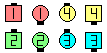Crazy Circulate\$B\$NA0(B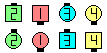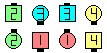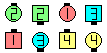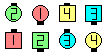Each Box Circulate(1/4)\$B\$N8e(B Center Box Circulate(1/2)\$B\$N8e(B Each Box Circulate(3/4)\$B\$N8e(B Center Box Circulate\$B\$N8e(B (\$B=*\$o\$j(B)

Crazy Counter Rotate 1/4: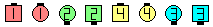Crazy Counter Rotate 1/4\$B\$NA0(B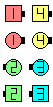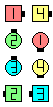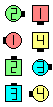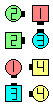Each SideCounter Rotate 1/4(1/4)\$B\$N8e(B CentersCounter Rotate 1/4(1/2)\$B\$N8e(B Each SideCounter Rotate 1/4(3/4)\$B\$N8e(B CentersCounter Rotate 1/4\$B\$N8e(B (\$B=*\$o\$j(B)

1/2 Crazy 1/4 Thru: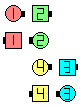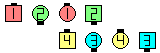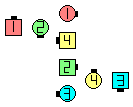1/2 Crazy 1/4 Thru\$B\$NA0(B Each side 1/4 Thru\$B\$N8e(B Centers 1/4 Thru\$B\$N8e(B (\$B=*\$o\$j(B)

Crazy Triangle Circulate: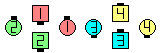Crazy Triangle Circulate\$B\$NA0(B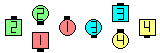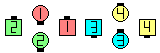Outside Triangles Circulate(1/4)\$B\$N8e(B Inside Triangles Circulate(1/2)\$B\$N8e(B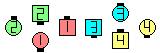Outside Triangles Circulate(3/4)\$B\$N8e(B Inside Triangles Circulate\$B\$N8e(B (\$B=*\$o\$j(B)

In the above example, Crazy Triangles means Outside Triangles do the call; Inside Triangles do the call; Outside Triangles do the call; Inside Triangles do the call.

\$B(assuming Parallel Waves or Columns):

• 3/4 Crazy Circulate:
Split Circulate; Centers Box Circulate; Split Circulate.
• Crazy Circulate 3:
Split Circulate 3 spots; Centers Box Circulate 3 spots; Split Circulate 3 spots; Centers Box Circulate 3 spots.
On Crazy Circulates, the caller can optionally give a series of 4 numbers indicating the number of Circulates to do at each part. For example, a Crazy Circulate 1-2-2-3 would be each side Circulate once; Centers Circulate twice; each side Circulate twice; Centers Circulate 3 times.

Reverse Crazy CONCEPT [C2]: Centers do the given call; each side do the given call; Centers do the given call; each side do the given call. This is a 4-part concept.CALLERLAB definition for Crazy ConceptChoreography for Crazy ConceptComments? Questions? Suggestions?